# Iterating over rows and columns in Pandas DataFrame

## Iterating over rows and columns in Pandas DataFrame

Iteration is a general term for taking each item of something, one after another. Pandas DataFrame consists of rows and columns so, in order to iterate over dataframe, we have to iterate a dataframe like a dictionary. In a dictionary, we iterate over the keys of the object in the same way we have to iterate in dataframe.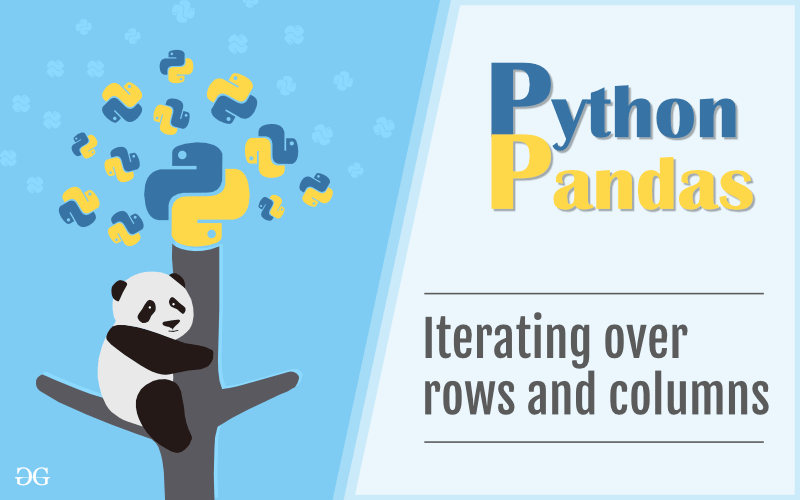In Pandas Dataframe we can iterate an element in two ways:

• Iterating over rows
• Iterating over columns

### Iterating over rows :

In order to iterate over rows, we can use three function iteritems(), iterrows(), itertuples() . These three function will help in iteration over rows.

#### Iteration over rows using iterrows()

In order to iterate over rows, we apply a iterrows() function this function returns each index value along with a series containing the data in each row.

Code #1:

 `# importing pandas as pd` `import` `pandas as pd` ` ` `# dictionary of lists` `dict` `=` `{``'name'``:[``"aparna"``, ``"pankaj"``, ``"sudhir"``, ``"Geeku"``],` `        ``'degree'``: [``"MBA"``, ``"BCA"``, ``"M.Tech"``, ``"MBA"``],` `        ``'score'``:[``90``, ``40``, ``80``, ``98``]}` `# creating a dataframe from a dictionary` `df ``=` `pd.DataFrame(``dict``)` `print``(df)`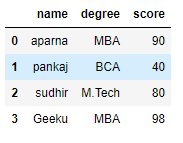Now we apply iterrows() function in order to get a each element of rows.

 `# importing pandas as pd` `import` `pandas as pd` ` ` `# dictionary of lists` `dict` `=` `{``'name'``:[``"aparna"``, ``"pankaj"``, ``"sudhir"``, ``"Geeku"``],` `        ``'degree'``: [``"MBA"``, ``"BCA"``, ``"M.Tech"``, ``"MBA"``],` `        ``'score'``:[``90``, ``40``, ``80``, ``98``]}` `# creating a dataframe from a dictionary` `df ``=` `pd.DataFrame(``dict``)` `# iterating over rows using iterrows() function` `for` `i, j ``in` `df.iterrows():` `    ``print``(i, j)` `    ``print``()`

Output: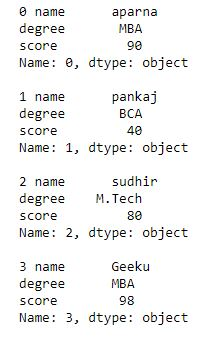Code #2:

 `# importing pandas module` `import` `pandas as pd` `   ` `# making data frame from csv file` `data ``=` `pd.read_csv(``"nba.csv"``)` `# for data visualization we filter first 3 datasets` `data.head(``3``)`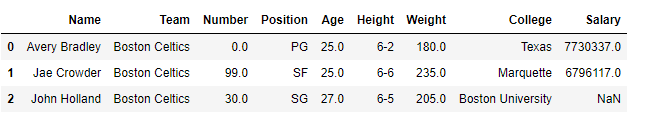Now we apply a iterrows to get each element of rows in dataframe

 `# importing pandas module` `import` `pandas as pd` `   ` `# making data frame from csv file` `data ``=` `pd.read_csv(``"nba.csv"``)` `for` `i, j ``in` `data.iterrows():` `    ``print``(i, j)` `    ``print``()`

Output: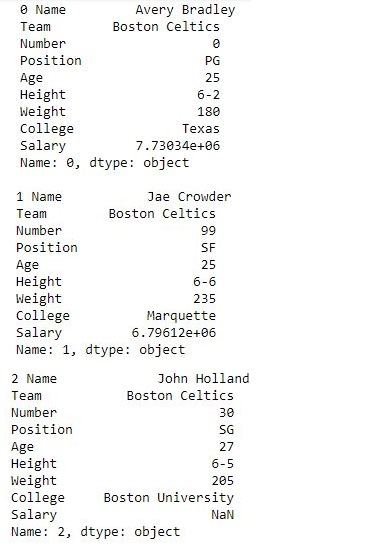#### Iteration over rows using iteritems()

In order to iterate over rows, we use iteritems() function this function iterates over each column as key, value pair with the label as key, and column value as a Series object.

Code #1:

 `# importing pandas as pd` `import` `pandas as pd` ` ` `# dictionary of lists` `dict` `=` `{``'name'``:[``"aparna"``, ``"pankaj"``, ``"sudhir"``, ``"Geeku"``],` `        ``'degree'``: [``"MBA"``, ``"BCA"``, ``"M.Tech"``, ``"MBA"``],` `        ``'score'``:[``90``, ``40``, ``80``, ``98``]}` `# creating a dataframe from a dictionary` `df ``=` `pd.DataFrame(``dict``)` `print``(df)`Now we apply a iteritems() function in order to retrieve an rows of dataframe.

 `# importing pandas as pd` `import` `pandas as pd` ` ` `# dictionary of lists` `dict` `=` `{``'name'``:[``"aparna"``, ``"pankaj"``, ``"sudhir"``, ``"Geeku"``],` `        ``'degree'``: [``"MBA"``, ``"BCA"``, ``"M.Tech"``, ``"MBA"``],` `        ``'score'``:[``90``, ``40``, ``80``, ``98``]}` `# creating a dataframe from a dictionary` `df ``=` `pd.DataFrame(``dict``)` `# using iteritems() function to retrieve rows` `for` `key, value ``in` `df.iteritems():` `    ``print``(key, value)` `    ``print``()`

Output: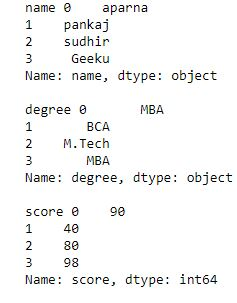Code #2:

 `# importing pandas module` `import` `pandas as pd` `   ` `# making data frame from csv file` `data ``=` `pd.read_csv(``"nba.csv"``)` `# for data visualization we filter first 3 datasets` `data.head(``3``)`

Output:Now we apply a iteritems() in order to retrieve rows from a dataframe

 `# importing pandas module` `import` `pandas as pd` `   ` `# making data frame from csv file` `data ``=` `pd.read_csv(``"nba.csv"``)` `for` `key, value ``in` `data.iteritems():` `    ``print``(key, value)` `    ``print``()`

Output: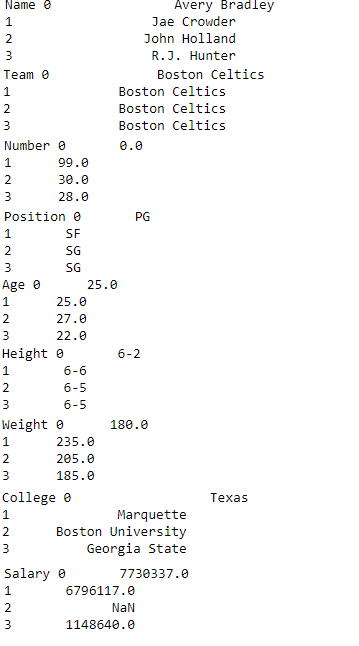#### Iteration over rows using itertuples()

In order to iterate over rows, we apply a function itertuples() this function return a tuple for each row in the DataFrame. The first element of the tuple will be the row’s corresponding index value, while the remaining values are the row values.

Code #1:

 `# importing pandas as pd` `import` `pandas as pd` ` ` `# dictionary of lists` `dict` `=` `{``'name'``:[``"aparna"``, ``"pankaj"``, ``"sudhir"``, ``"Geeku"``],` `        ``'degree'``: [``"MBA"``, ``"BCA"``, ``"M.Tech"``, ``"MBA"``],` `        ``'score'``:[``90``, ``40``, ``80``, ``98``]}` `# creating a dataframe from a dictionary` `df ``=` `pd.DataFrame(``dict``)` `print``(df)`Now we apply a itertuples() function inorder to get tuple for each row

 `# importing pandas as pd` `import` `pandas as pd` ` ` `# dictionary of lists` `dict` `=` `{``'name'``:[``"aparna"``, ``"pankaj"``, ``"sudhir"``, ``"Geeku"``],` `        ``'degree'``: [``"MBA"``, ``"BCA"``, ``"M.Tech"``, ``"MBA"``],` `        ``'score'``:[``90``, ``40``, ``80``, ``98``]}` `# creating a dataframe from dictionary` `df ``=` `pd.DataFrame(``dict``)` `# using a itertuples()` `for` `i ``in` `df.itertuples():` `    ``print``(i)`

Output: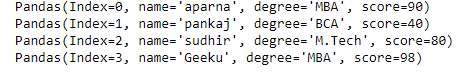Code #2:

 `# importing pandas module` `import` `pandas as pd` `   ` `# making data frame from csv file` `data ``=` `pd.read_csv(``"nba.csv"``)` `# for data visualization we filter first 3 datasets` `data.head(``3``)`Now we apply an itertuples() to get atuple of each rows

 `# importing pandas module` `import` `pandas as pd` `   ` `# making data frame from csv file` `data ``=` `pd.read_csv(``"nba.csv"``)` `for` `i ``in` `data.itertuples():` `    ``print``(i)`

Output: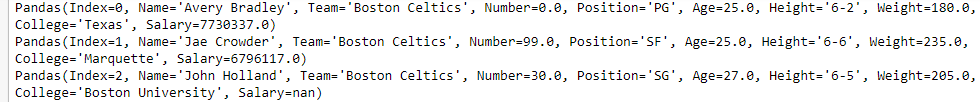### Iterating over Columns :

In order to iterate over columns, we need to create a list of dataframe columns and then iterating through that list to pull out the dataframe columns.

Code #1:

 `# importing pandas as pd` `import` `pandas as pd` `  ` `# dictionary of lists` `dict` `=` `{``'name'``:[``"aparna"``, ``"pankaj"``, ``"sudhir"``, ``"Geeku"``],` `        ``'degree'``: [``"MBA"``, ``"BCA"``, ``"M.Tech"``, ``"MBA"``],` `        ``'score'``:[``90``, ``40``, ``80``, ``98``]}` ` ` `# creating a dataframe from a dictionary` `df ``=` `pd.DataFrame(``dict``)` `print``(df)`Now we iterate through columns in order to iterate through columns we first create a list of dataframe columns and then iterate through list.

 `# creating a list of dataframe columns` `columns ``=` `list``(df)` `for` `i ``in` `columns:` `    ``# printing the third element of the column` `    ``print` `(df[i][``2``])`

Output: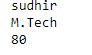Code #2:

 `# importing pandas module` `import` `pandas as pd` `   ` `# making data frame from csv file` `data ``=` `pd.read_csv(``"nba.csv"``)` `# for data visualization we filter first 3 datasets` ` ``col ``=` `data.head(``3``)` `col`Now we iterate over columns in CSV file in order to iterate over columns we create a list of dataframe columns and iterate over list

 `# creating a list of dataframe columns` `clmn ``=` `list``(col)` `for` `i ``in` `clmn:` `    ``# printing a third element of column` `    ``print``(col[i][``2``])`

Output: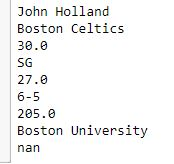Last Updated on October 24, 2021 by admin

## Python | Pandas Timedelta.daysPython | Pandas Timedelta.days

Python | Pandas Timedelta.days Python is a great language for doing data analysis, primarily because

## Set Pandas dataframe background Color and font color in PythonSet Pandas dataframe background Color and font color in Python

Set Pandas dataframe background Color and font color in Python As we know, the basic

## Convert A Categorical Variable Into Dummy VariablesConvert A Categorical Variable Into Dummy Variables

Convert A Categorical Variable Into Dummy Variables All the statistical and machine learning models are

## Pandas Series.rename()Pandas Series.rename()

Python | Pandas Series.rename() Pandas series is a One-dimensional ndarray with axis labels. The labels

## Python | Pandas dataframe.max()Python | Pandas dataframe.max()

Pandas dataframe.max() Pandas dataframe.max() function returns the maximum of the values in the given object.

## Creating A Time Series Plot With Seaborn And PandasCreating A Time Series Plot With Seaborn And Pandas

Creating A Time Series Plot With Seaborn And Pandas In this article, we will learn

## Check if a column starts with given string in Pandas DataFrame?Check if a column starts with given string in Pandas DataFrame?

Check if a column starts with given string in Pandas DataFrame? In this program, we

## Python – Convert Tick-by-Tick data into OHLC (Open-High-Low-Close) DataPython – Convert Tick-by-Tick data into OHLC (Open-High-Low-Close) Data

Python – Convert Tick-by-Tick data into OHLC (Open-High-Low-Close) Data In this post, we’ll explore a